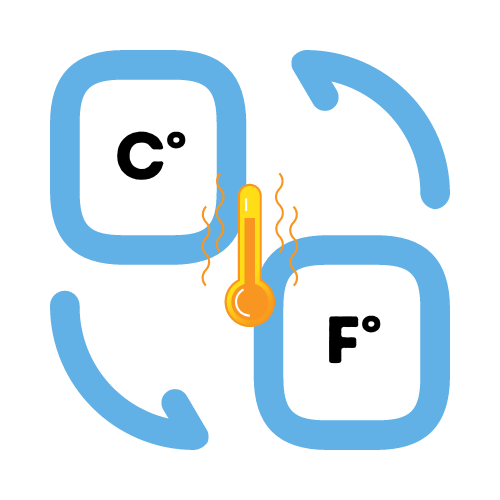# Convert Celsius to Fahrenheit

## Temperature conversion from Celsius to Fahrenheit.

• Created by Emily Rodriguez
• Reviewed by Ramesh Agarwal
Enter a number.

Temperature in Fahrenheit:

View all Unit Conversion Calculators

## How to use the temperature converter

You can add a temperature in celsius and quickly get the results returned in farenheit. The calculator will convert automatically swap the values for you.

You can also convert temperatures the other direction by visiting the fahrenheit to celsius converter.

## How do you convert Celsius to Fahrenheit?

The conversion between celsius and fahrenheit is rather interesting. To convert celsius to fahrenheit, you need to take the initial celsius value and multiply by 9/5, then add 32 to the result.

Example conversion for 30 degrees:
`30 degree celsius = 30 * (9 / 5) + 32 = 86° farenheit`

## How do I convert a temperature from Celsius to Fahrenheit without a calculator?

To convert a temperature from Celsius to Fahrenheit in your head, you can use the following "rule of thumb": multiply the Celsius temperature by 2, then add 30. This will give you an approximation of the temperature in Fahrenheit.

## What is the Celsius to Fahrenheit conversion formula?

The formula for converting Celsius to Fahrenheit is:
`Fahrenheit = Celsius * 1.8 + 32`

## How do I convert a temperature from Fahrenheit to Celsius?

To convert a temperature from Fahrenheit to Celsius, use the following formula:
`Celsius = (Fahrenheit - 32) / 1.8`

## How do I convert a temperature from Fahrenheit to Celsius in my head?

To convert a temperature from Fahrenheit to Celsius in your head, you can use the following "rule of thumb": subtract 30 from the Fahrenheit temperature, then divide the result by 2. This will give you an approximation of the temperature in Celsius.

## Fahrenheit to Celsius Conversion Table

This table shows values in Fahrenheit and their equivalent values in Celsius.

Celsius Fahrenheit
-50 -58
-40 -40
-30 -22
-20 -4
-10 14
0 32
10 50
20 68
30 86
40 104
50 122